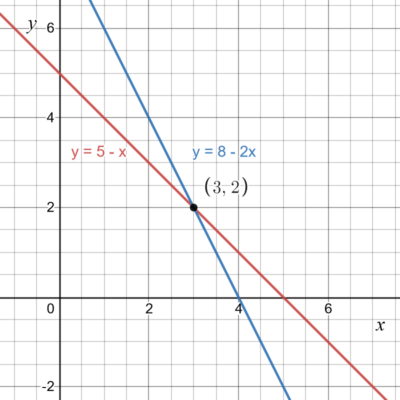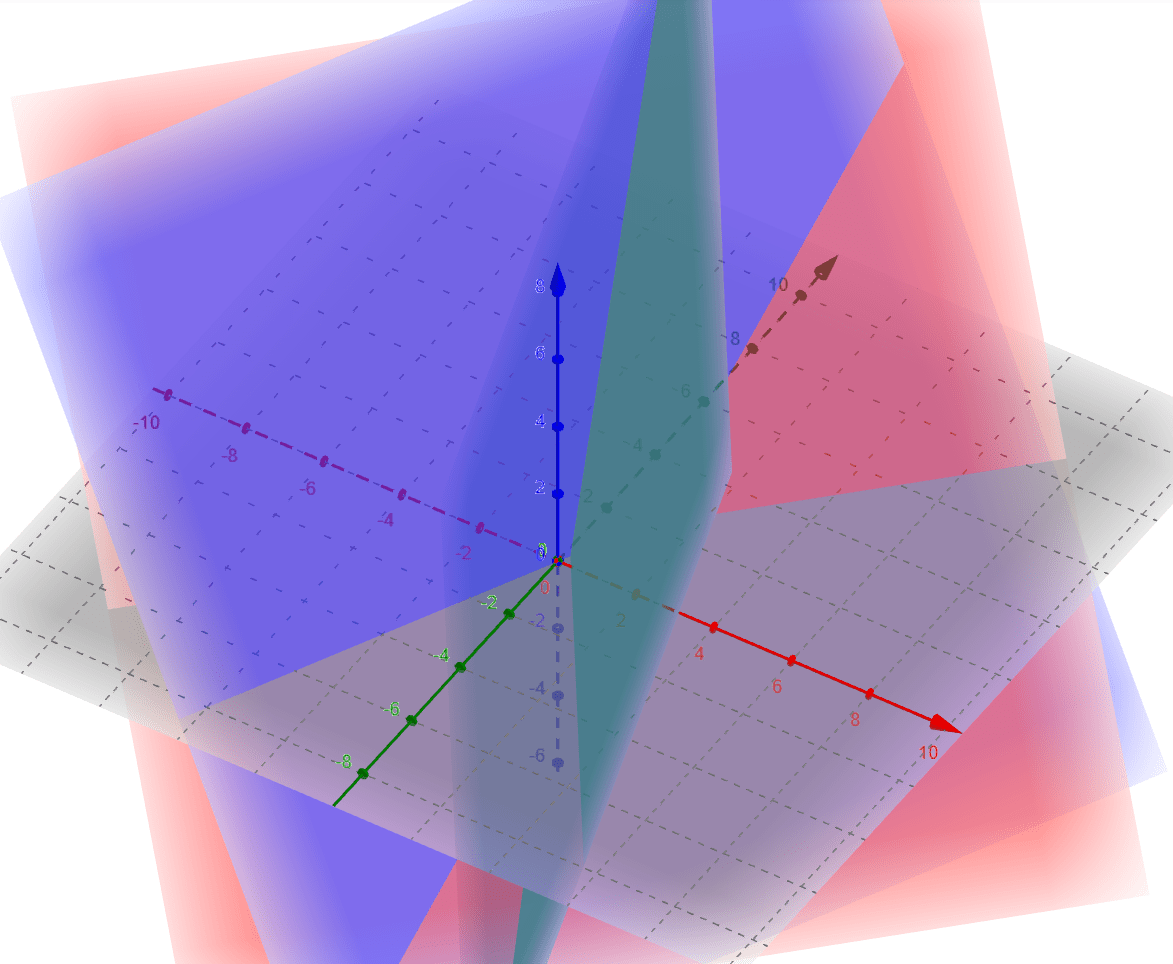Introduction to System of Linear Equations

What is a system of linear equations? A system of linear equations is a set of equations with more than one unique variable. There can be either no solution, one unique solution or multiple solutions for the system. For now, we will assume that all examples will have strictly one unique solution. For example,$x + y = 5$$2x + y = 8$

is a system of 2 equations with variables$x$ and$y$. When solving this, you must consider all of the equations involved and find a solution that satisfies all of both equations. In NMDev, these equations can be represented with AbstractRealScalarFunction, AbstractBivariateRealFunction and AbstractTrivariateRealFunction. AbstractBivariateRealFunction is predefined function for an equation with 2 unique variables, while the AbstractTrivariateRealFunction is predefined for 3 unique variables. Meanwhile, for the AbstractRealScalarFunction, you will have to specify the number of variables when you are initialising the function.

We can define a function$f(x,y)=x+y-5$ in NMDev as such

				
%use s2
//define function x + y = 5 with RealScalarFunction
val f1:RealScalarFunction = object : AbstractRealScalarFunction(2) {
//setting the value as 2 for the 2 variables
override fun evaluate(v: Vector): Double {
val x:Double = v.get(1)
val y:Double = v.get(2)
//if the values are correct, it returns 0
return x + y - 5
}}
//calling f1 with vector of x = 2, y = 3
println(f1.evaluate(DenseVector(arrayOf(2.0,3.0))))

//define function x + y = 5 with AbstractBivariateRealFunction
val f2:BivariateRealFunction = object : AbstractBivariateRealFunction() {
override fun evaluate(x: Double, y:Double): Double {
//AbstractBiRealFunction recieves two variables as the input
//if the values are correct, it returns 0
return x + y - 5
}}
//calling f2 with values x = 2, y = 3
println(f2.evaluate(2.0,3.0))



While representing an equation of 3 variables$x+y+z=10$ would look like this

				
%use s2
//define function x + y + z = 10 with RealScalarFunction
val f1:RealScalarFunction = object : AbstractRealScalarFunction(3) {
//setting the value as 3 for the 3 variables x, y and z
override fun evaluate(v: Vector): Double {
val x:Double = v.get(1)
val y:Double = v.get(2)
val z:Double = v.get(3)
//if the values are correct, it returns 0
return x + y + z - 10
}}
//calling f1 with vector of x = 2, y = 3, z = 5
println(f1.evaluate(DenseVector(arrayOf(2.0,3.0,5.0))))

//define function x + y + z = 10 with AbstractTrivariateRealFunction
val f2:TrivariateRealFunction = object : AbstractTrivariateRealFunction() {
override fun evaluate(x: Double, y:Double, z:Double): Double {
//AbstractTrivariateRealFunction recieves three variables as input
//if the values are correct, it returns 0
return x + y + z - 10
}}
//calling f2 with values x = 2, y = 3, z = 5
println(f2.evaluate(2.0,3.0,5.0))




Food For Thought:

It would also be possible to represent a system of linear equations as a matrix, with the rows representing the equation and columns representing the variables. How would you represent the same systems shown above as a matrix in NMdev?

Graphing Method

A way to visualize a system of linear equations is with the use of a graph. Using this system,$y = 5-x$$y = 8-2x$

we can represent it in the following graph.From the above graphs, you can see that the$x$ and$y$ values where both equations will be fulfilled is at the point of intersection, which is$x = 3$,$y = 2$. To prove that the solution is valid, you can substitute$x = 3$ and$y = 2$,$2 = 5-3$$2 = 8-3*2$

Hence, the system of equations is valid when$x = 3$,$y = 2$.

System of 3 or more variables

There can be more than just 2 variables in a system of equations. For example,$3x+2y-z=1$$2x-2y+4z=-2$$-x+1/2 y-z=0$

is a system of 3 equations with 3 variables,$x$,$y$ and$z$. In a system with 3 variables, you can use a 3D graph to visualize the 3 equations using$x$,$y$ and$z$ as the vertices. Each equation is represented as its own plane, and the intersection of two of the planes would be a straight line. The intersections of the planes would meet at a single point, resulting in a single set of values which is the solution for the system of equations.This would be a visualisation of the system, with the point of intersection being the solution. In this case,$x=1$$y=-2$$z=-2$

is the solution to the system. This is because the point of intersection is the only point where the values of$x$,$y$ and$z$ holds true for all the equations in the system. In essence, no matter the number of variables, you are able to determine if a set of numbers is the solution if all equations in a system of equations holds true with the set of numbers.

### Real World Examples!

System of Equations commonly occur in real world scenarios! One of the most common examples of this is when comparing costs of 2 different electricity companies.

Assume that company A costs $0.10 per watt while company B costs$0.05 per watt with initial set-up cost of \$100. Can you use system of equations to determine how many watts must you use before the costs of using company B equal to that of using company A?

Alternate solutions

Generally, there are three ways a system of linear equations can behave.

1. The system has a single unique solution. (All graphs intersects at one unique point)
2.  The system has infinitely many solutions. (All graphs of the equations are on the same line)
3. The system has no solution. (All graphs do not intersect at any point)

We have thus far been working with the assumption that the system follows the first behavior mentioned for all equations, but there are other solutions that you have to take note. For the below examples, we will use 2 variables,$x$ and$y$, to showcase the different solutions of systems.

Infinitely many solutions

A system which has infinitely many solutions is a system where all the equations are valid at every possible value. Graphically, the equations will be on the same line. For example,$y=5-x$$2y=10-2x$

is a system where the both equations share the same line. The graph will look like this.With this system, there will be infinite solutions as there is a point of intersection for each and every value of$x$, hence infinite values of$x$ can be the solution.

No solutions

A system which has no solutions is a system in which there are no values for the variables where the equations will be valid. Graphically, there will be no point of intersection where all the graphs pass through. For example,$y=2x+3$$y=2x+5$$y=-x+1$

is a system which will have no solutions as there is no point at which all 3 equations intersect.With this system, there is no solutions as there is no point on the graph where the$x$ and$y$ values are constant for all equations in the system.